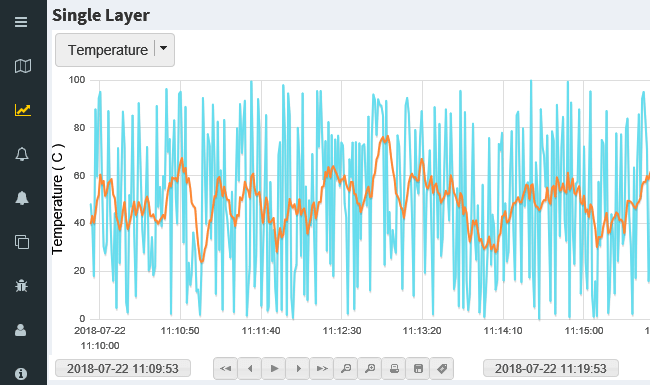##### How to calculate Moving Averages in SCADA?

Mr. Wachira has recently asked how to do moving averages in SCADA, that made us recall how troublesome is to do some simple calculations in traditional SCADA. It can be even more painful when you need to deal with proprietary programming language. As such we are more than happy to share how elegant to calculate moving averages in IGX Web SCADA using standard JavaScript.The trending showing two lines from same source, but one has applied with moving average, and you know exactly which one.

Below is the code listing which is completely self explanatory with 100% comment. Only thing to note is that `getTag()` & `setTag()` is the external functions for fetching and storing data from and to your database or real-time system.

```//set a constant for total sample, the bigger the more stable but less reactive
var MAX = 10;

//simulate raw input data.
var data = Math.random() *100;

//fetch list of raw data that previously stored in string
var list = getTag( "avg_list") || "";

//convert string to array
var a = list.split(",");

//push in new raw data into array
a.push( data);

//magic code sums up values in array, otherwise a loop is needed.
var sum = a.reduce( function(x, y) {return (+x) + (+y);}, 0);

//variable length to cater for initial values.
var l = a.length;

//Result is sum divide by sample quantity, default 1 to avoid 0 div err.
setTag( "avg_data", (sum/l) || 1);

//shift out oldest data
if (l > MAX) {
a.shift();
}

//store array back into string
setTag( "avg_list", a +"");

```

Do you have any inquiry which we should share too?